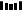## 初识广度优先搜索

class ListNode {
int val;
ListNode next;
}


class TreeNode {
int val;
TreeNode left, right;
}


class TreeNode {
int val;
List<TreeNode> children;
}


class GraphNode {
int val;
List<Integer> neighbors;
}


• 层级遍历
• 由点到面遍历图
• 拓扑排序
• 求最短路径

## 二叉树的锯齿形层次遍历

### 题目描述

    3
/
9  20
/
15   7


[
,
[20,9],
[15,7]
]


### 参考代码

public List<List<Integer>> zigzagLevelOrder(TreeNode root) {
List<List<Integer>> result = new ArrayList<>();

if (root == null) {
return result;
}

queue.offer(root);

boolean isReverse = false;
while (!queue.isEmpty()) {
int size = queue.size();

List<Integer> curLevel = new ArrayList<>();

for (int i = 0; i < size; ++i) {
TreeNode cur = queue.poll();
if (cur.left != null) {
queue.offer(cur.left);
}

if (cur.right != null) {
queue.offer(cur.right);
}

if (isReverse) {
} else {
}
}

isReverse = !isReverse;

}

return result;
}


## 以图判树

### 题目描述

输入: n = 5, 边列表 edges = [[0,1], [0,2], [0,3], [1,4]]



输入: n = 5, 边列表 edges = [[0,1], [1,2], [2,3], [1,3], [1,4]]



### 参考代码

public boolean validTree(int n, int[][] edges) {
if (n - 1 != edges.length) {
return false;
}

Map<Integer, Set<Integer>> graph = new HashMap<>();

buildGraph(graph, edges, n);

Set<Integer> visited = new HashSet<>();

queue.offer(0);

while (!queue.isEmpty()) {
int cur = queue.poll();

return false;
}

for (int nei : graph.get(cur)) {
queue.offer(nei);

graph.get(nei).remove(cur);
}
}

return visited.size() == n;
}

private void buildGraph(Map<Integer, Set<Integer>> graph, int[][] edges, int n) {
for (int i = 0; i < n; ++i) {
graph.put(i, new HashSet<Integer>());
}

for (int[] edge : edges) {
}
}


## 总结1.程序员

2.【GitHub

3.【算法动画：七分钟理解什么是KMP算法

4.【数据结构十大经典排序算法动画与解析，看我就够了！